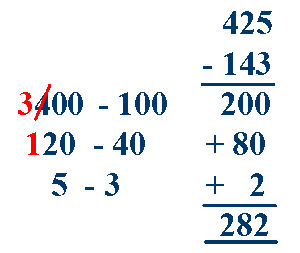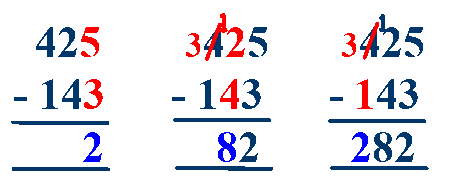# Written methods of Subtraction

## Estimate and Check

Before writing out a calculation it is a good idea to make an estimate first. This is a simple and quick process which helps to ensure that you do not make silly mistakes.

Say you need to subtract 97 from 402 - simply round the first number 402 to 400, then round the second number 97 to 100 - subtract the two rounded numbers, 400 - 100 = 300 and you now know that your final answer should be close to 300. When you have completed your calculation, check that it is similar to your estimate and that it makes sense.

## Splitting Method

This method involves partitioning the numbers into 100's, 10's and units, adding these individually and then combining the results to give a final answer.

You can do this by splitting across.

When one of the columns has a larger number on the bottom (40 > 20 in the tens) then you need to borrow from the column to its left.
So we use one of the hundreds from the hundreds column - 400 becomes 300 - and 20 becomes 120.

#####or by splitting down.

Again, one of the columns has a larger number on the bottom (40 > 20 in the tens) then you need to borrow from the column to its left.
So we use one of the hundreds from the hundreds column - 400 becomes 300 - and 20 becomes 120.Yet again this method shows clearly what is happening when two numbers are subtracted and is great for understanding the process - however it takes time and quite a lot of writing.

## Standard Method

This is the preferred method and requires a good understanding of place value, how to partition numbers, be able to subtract multiples of 10 and to know addition and subtraction facts up to 20. It is important to keep the numbers in the correct columns (using lined paper sideways, so that the lines are vertical, helps with this).

First subtract the units, 5 - 3 = 2, put the 2 in the units column below the question.

Next subtract the tens, 2 - 4 does not give a positive answer so we need to borrow from the column to its left. The 2 in the tens column becomes 12 and the 4 in the hundreds column becomes 3 - now we can subtract the tens, 12 - 4 = 8, put the 8 in the tens column below the question.

Next subtract the hundreds, 3 - 1 = 2, put the 2 in the hundreds column below the question.### Try the Subtraction Mission - Game 2

Full screen version